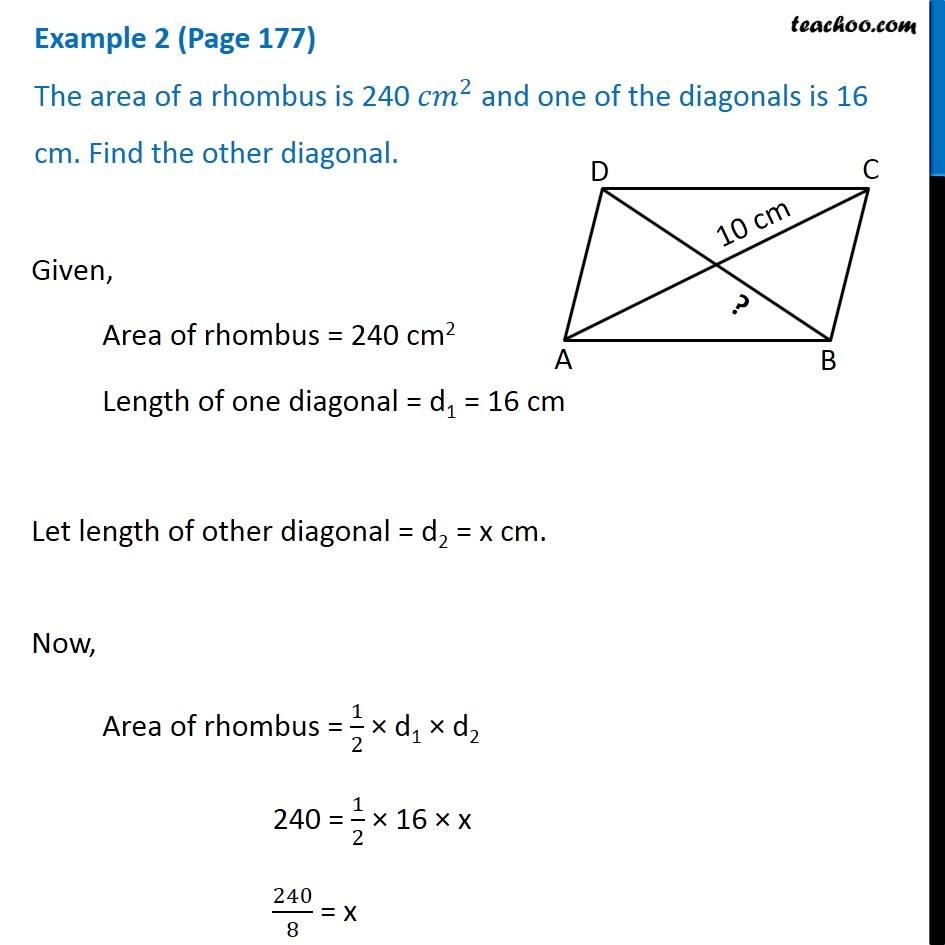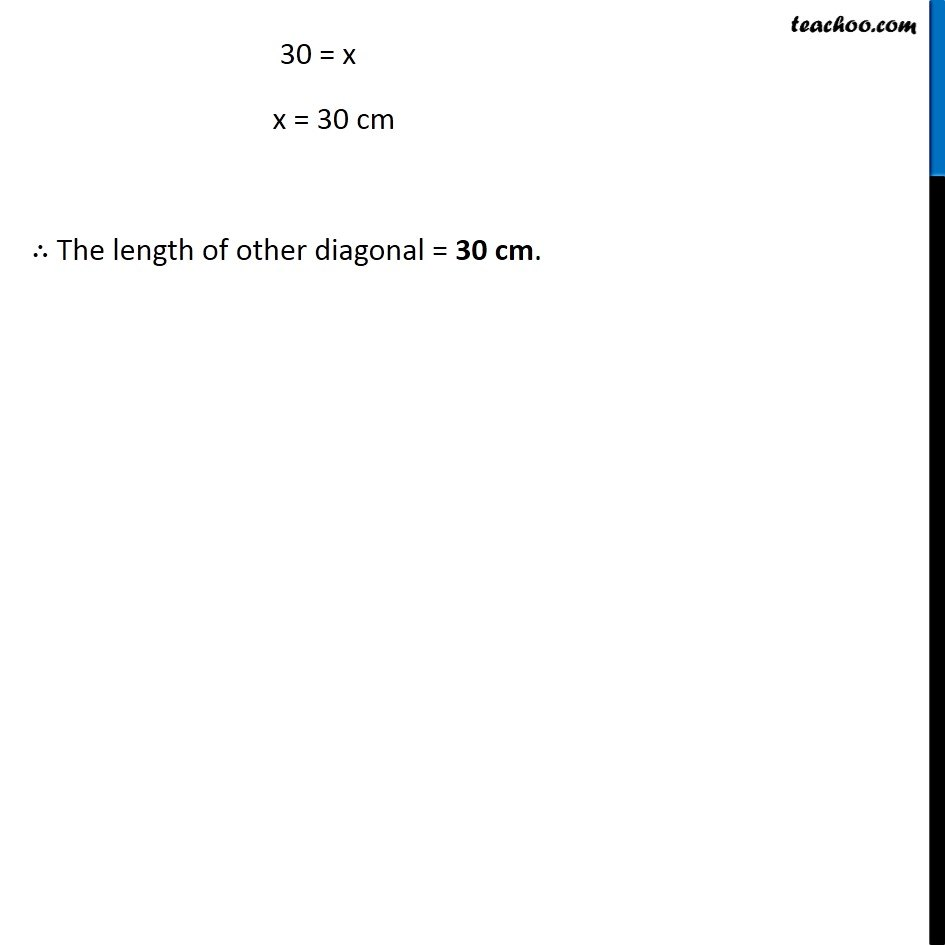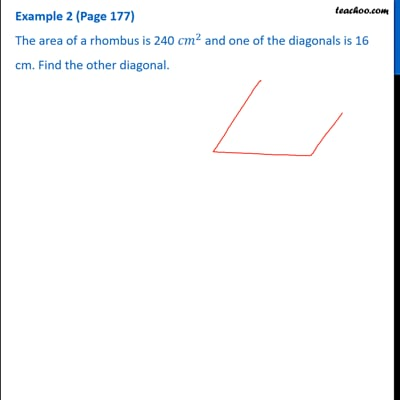Examples

Chapter 9 Class 8 Mensuration
Serial order wiseThis video is only available for Teachoo black users

Learn in your speed, with individual attention - Teachoo Maths 1-on-1 Class

### Transcript

Example 2 (Page 177) The area of a rhombus is 240 〖𝑐𝑚〗^2 and one of the diagonals is 16 cm. Find the other diagonal. Given, Area of rhombus = 240 cm2 Length of one diagonal = d1 = 16 cm Let length of other diagonal = d2 = x cm. Now, Area of rhombus = 1/2 × d1 × d2 240 = 1/2 × 16 × x 240/8 = x 30 = x x = 30 cm ∴ The length of other diagonal = 30 cm.